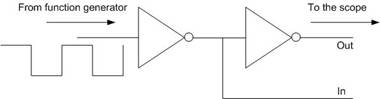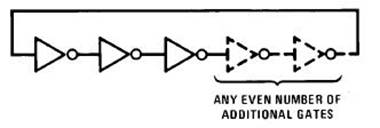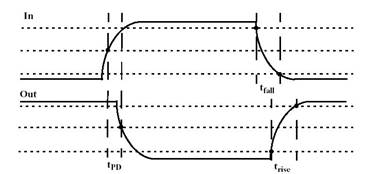Peter Lau

Bill Chun Wai Hung

Lab 14 Report – Propagation Delay and Inverter Ring Oscillator

9 August 2004

A. Describe the Set-up

The Circuit 1 setup is shown in figure 1.Figure 1

The Circuit 2 setup is shown in figure 2.Figure 2Figure 3 – measure the time of the 10% and 90% points

B1. Describe Inputs

Stimuli:

DC 5V to drive the inverters

Inverters Chip: 74LS04

AC 5V square wave

C. Describe What you Observe

Circuit 1.

Detla t (2 inverters) = 15.40ns

Detla t (1 inverters) = 7.70ns

Rise Time = 9.80 ns

Fall Time = 7.80 ns

Circuit 2.

Oscillation Frequency = 24.91MHz

The propagation delay is (1/24.91MHz) / 6 = 6.69 ns

D. What you can deduce

For Circuit 1, the experimental propagation delay (7.70ns) agrees with the propagation delay stated in the data sheet (from 3ns to 10 ns).

The rise time measured between the 10% and 90% points of the input and output signal is 9.80ns. The fall time measured between the 10% and 90% points of the input and output signal is 7.80ns.

From Circuit 2,

The three inverters oscillate when connected in series. The oscillation frequency is 24.91MHz.

The propagation delay is (1/24.91MHz) / 6 = 6.69 ns

Divide the time by 6 because there are 3 inverters and rise and fall delay(2 delay).

Q1) Explain why the ring circuit generates oscillation.

Because the logic opposite is feedback to the input after certain time delay. This causes the first gate to flip and the reverse gate is propagated to the remainder of the chain, and this process repeats itself during the process of oscillation.

Q2) Is the propagation delay the same for the two experiment?

Yes, they are the same. The propagation delay for circuit 1 is 7.70ns and that of circuit 2 is 6.69ns, very close to be the same.

Q3) If not, explain what could be the possible causes.

The wire connections of the circuit can cause extra delays.